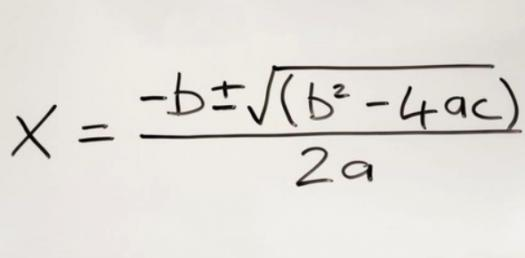# Quadratic Equations Practice Test 1

10 Questions | Total Attempts: 2960Settings• 1.
-1x2 + 0x + 49 = 0
• A.

X = -9 and -6

• B.

X = 7 and -7

• C.

X = 8 and 3

• D.

X = 7 and -3

• E.

X = 9 and -9

• 2.
-1x2 + 2x + 48 = 0
• A.

X = -2 and 1

• B.

X = -1 and -7

• C.

X = 9 and -9

• D.

X = 8 and -6

• E.

X = 8 and -6

• 3.
1x2 + 5x - 14 = 0
• A.

X = -1 and 2

• B.

X = -1 and 2

• C.

X = -7 and 2

• D.

X = 9 and -9

• E.

X = 9 and -9

• 4.
1x2 + 10x + 21 = 0
• A.

X = -7 and -3

• B.

X = -7 and -3

• C.

X = 8 and -6

• D.

X = 8 and 6

• E.

X = 10 and 11

• 5.
-1x2 + 3x + 28 = 0
• A.

X = -6 and -8

• B.

X = 9 and 4

• C.

X = 6 and -5

• D.

X = -7 and -4

• E.

X = 7 and -4

• 6.
What is the vertex of the following equation: x2 - 8x + 15 = 0?
• A.

(4,1)

• B.

(4,-1)

• C.

(-4,-1)

• D.

(-4,1)

• 7.
What is the  axis of symmetry and range of the following function: x2 - 8x + 15 = 0?
• A.

Axis: x=4 ; Range: (-1,infinity)

• B.

Axis: x=-4 ; Range: (-1, infinity)

• C.

Axis: x=-1 ; Range: (4, infinity)

• D.

Axis: x=-1 ; Range: (-4, infinity)

• 8.
What is the vertex of the following equation:  -x2 - 9x - 8 = 0?
• A.

(1,-9)

• B.

(-1,-9)

• C.

(-1,9)

• D.

(1,9)

• 9.
What is the range of the following function:  -x2 + 2x + 8 = 0?
• A.

(infinity, 9)

• B.

(-infinity, infinity)

• C.

(9, infinity)

• D.

(-9, infinity)

• E.

(-infinity, 9)

• 10.
What is the domain of the following function:  -x2 + 2x + 8 = 0?
• A.

(1,9)

• B.

(-infinity,infinity)

• C.

(infinity,9)

• D.

(1, infinity)

• E.

(9,infinity)

Related TopicsBack to top